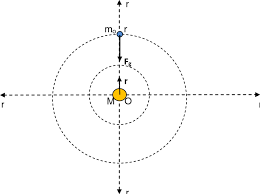## How to Calculate and Solve for Gravitational Force | The Calculator EncyclopediaThe image above represents the gravitational force.

To compute the gravitational force of a field, three parameters are needed and this parameters are mass (m1), mass (m2) and radius between the masses (R).

The formula for calculating the gravitational force:

F = Gm1m2 /

Where;
F = Gravitational force
m1 = Mass 1
m2 = Mass 2
r = Radius between the masses

Let’s solve an example;
Find the gravitational force of a field when the mass 1 is 8 cm, mass 2 is 10 cm and the radius between masses is 14 cm.

This implies that;
m1 = Mass 1 = 8 cm
m2 = Mass 2 = 10 cm
r = Radius between the masses = 14 cm

F = Gm1m2 /
F = (6.67 x 10-11 x 8 x 10) / 196
F = 5.336e-9 / 196
F = 2.722e-11

Therefore, the gravitational force is 2.722e-11 Newton (N).